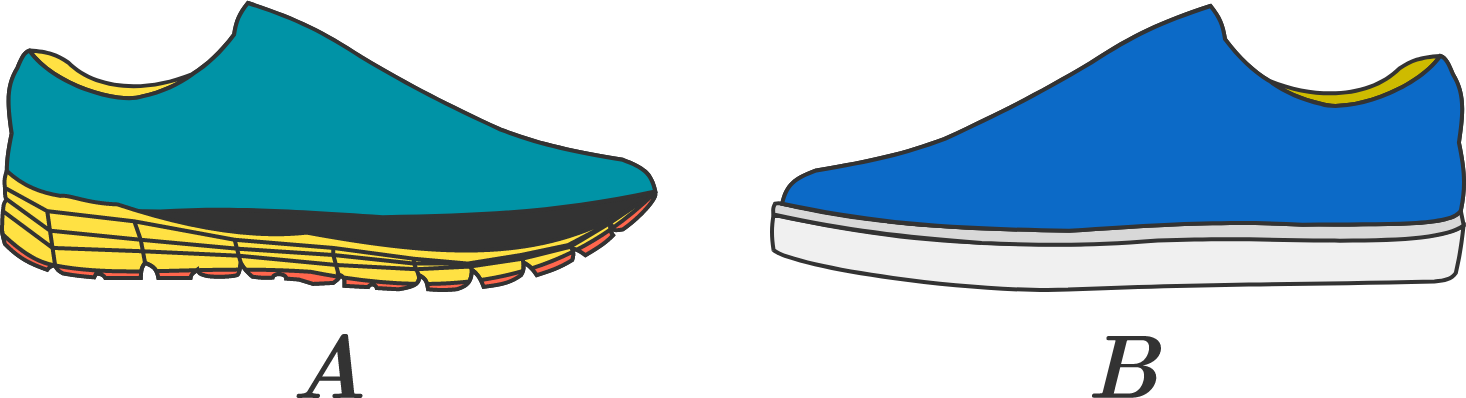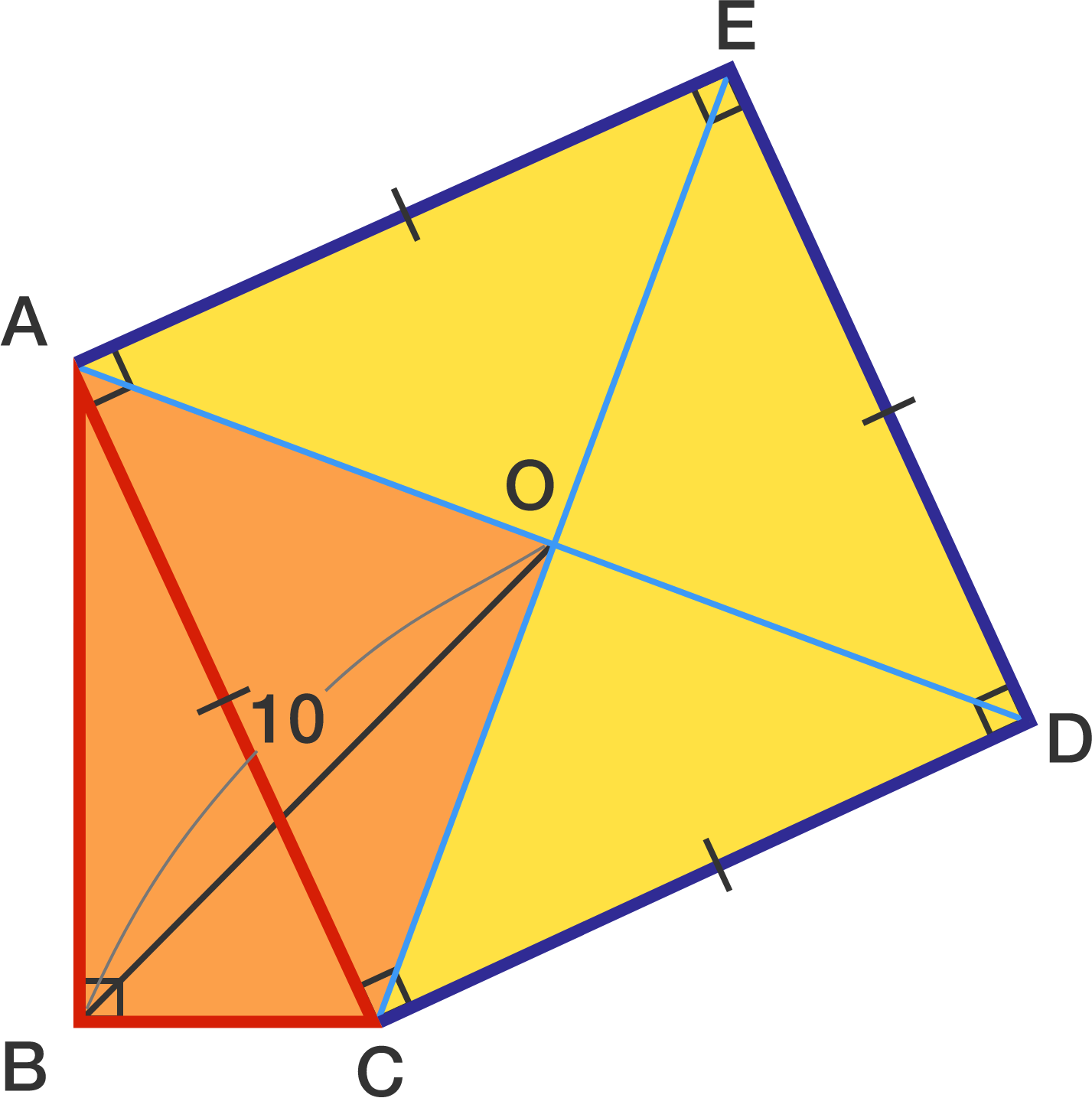# Problems of the Week

Contribute a problem

# 2017-02-27 IntermediateThere are 2 businessmen, who wear different types of shoes. Businessman $A$ wears athletic shoes with bumpy soles, while businessman $B$ wears dress shoes with smooth soles. Assume that both pairs of shoes are newly purchased (and standardized much like their own types).

They are both walking very quickly on a marble floor that is slightly wet as it was recently mopped. Which businessman is more prone to slip on the floor while walking?


Tip: It might be helpful to study the structures of the shoe soles before you approach the problem.In triangle $ABC$, $\angle ABC = 90 ^ \circ$.
Square $ACDE$ with center $O$ is drawn externally on side $AC$ of the triangle.
If $OB = 10$, what is the area of quadrilateral $ABCO ?$

Recall that
$e^x = 1 + \frac{x}{1!} + \frac{x^2}{2!} + \frac{x^3}{3!} + \cdots.$ Then what is the value of $\large \frac{\frac{2}{3!} + \frac{4}{5!} + \frac{6}{7!} + \cdots}{\frac{2}{1!} + \frac{4}{3!} + \frac{6}{5!} + \cdots}\, ?$


Notation: $!$ is the factorial notation. For example, $8! = 1\times2\times3\times\cdots\times8$.You have 4 stones. They are of four distinct colors, and they are also of distinct weights of 1, 2, 3, 4 grams. However, you do not know which weight corresponds to which color. The balance scale you have can only indicate which side is heavier or whether the two sides are balanced, without further details. You may put as many stones as you want on each side of the scale.

What is the least number of weighing trials needed to ensure correct weight labeling for all stones?

A jump jet of mass $\SI{400}{\kilogram}$ (without fuel) can hover, stationary, by burning fuel and blasting the waste products downwards at a constant velocity of $\SI[per-mode=symbol]{1000}{\meter \per \second}$ (relative to the jet). It is carrying $\SI{100}{\kilogram}$ of fuel.

How long, in seconds, can the jump jet hover like this before it runs out of the fuel?

Assume that the fuel contains all of the reactants: i.e. no air is being drawn from outside. (A real jump jet would take in air from outside, which would make up a substantial fraction of the exhaust mass.)

Take the acceleration due to gravity to be $\SI[per-mode=symbol]{10}{\meter \per \second \squared}$.